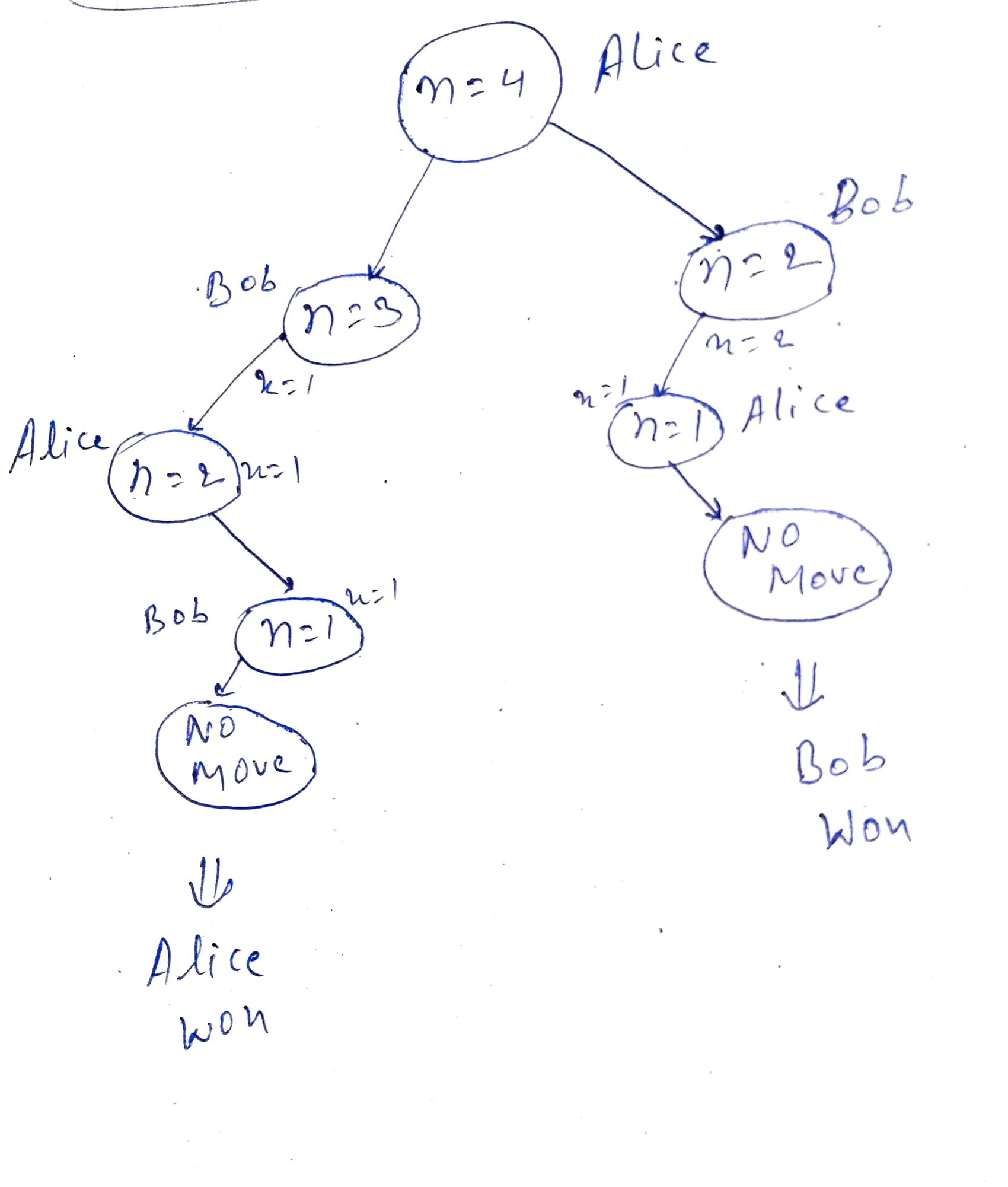Engineer bro!

Wed Nov 17 2021 (10 months ago)

Engineer by mistake!

I am a software engineer by passion

# Divisor Game a Dynamic programming problem to get started with DP

In this article, you'll learn that how can you use DP to reduce the execution time.

## Problem description

Alice and Bob take turns playing a game, with Alice starting first.

Try it

Initially, there is a number n on the chalkboard. On each player's turn, that player makes a move consisting of:

• Choosing any $XX$ with $0 and $n\mathrm{%}X==0n \% X == 0$.

• Replacing the number n on the chalkboard with n - x.

Also, if a player cannot make a move, they lose the game.

Return true if and only if Alice wins the game, assuming both players play optimally.

### Example 1

Input: n = 2
Output: true
Explanation: Alice chooses 1, and Bob has no more moves.


### Exmaple 2

Input: n = 3
Output: false
Explanation: Alice chooses 1, Bob chooses 1, and Alice has no more moves.


### Constraints

$1<=n<=10001 <= n <= 1000$

## Explanation

• Suppose you have a number $n=1n=1$. As per the problem description, Alice will start playing first. For $n=1n=1$, there is no x such that ($0), hence Alice will lose the game.

• Suppose, you have a number $n=2n=2$. First, Alice can choose $X=1X=1$ and make $n=1n=1$. Now, Bob has $n=1n=1$ and he can not make any move. So, Bob lose the game.

• Suppose, you have a number $n=4n=4$. This time Alice can choose either $11$ or $22$. Now the real DP game begains.

###### DP TreeWhen you see the above image, then you can see at each level either Alice or Bob is present.

Steps

1. Alice started the game, now he can choose either 1 or 2

2. Alice chooses 1

1. $n=3n=3$ and it's Bob's turn. He can choose 1 and n becomes 2

2. $n=2n=2$ and it's Alice's turn. He can choose 1 and n becomes 1

3. $n=1n=1$ and it's Bob's turn. He can not choose any x and Bob loses the game

3. Alice chooses 2

1. $n=2n=2$ and it's Bob's turn. He can choose 1 and n becomes 1

2. $n=1n=1$ and it's Alice's turn. He can not choose any x, so he loses the game.

You can observe that initially if Alice were not played optimally and choose 2 then Alice would have to lose.

## Brute force method

From the above explanation, you can see that at each step you have to make a decision. The decision should be optimal, so the current player wins the game.

You are given a number $nn$ and you have to return true if Alice will win the game or false.

Alice can win the game if and only if there is a possibility where can Bob loses the game.

1. Let's $XX$ is a set of number which can divide $nn$ and $0.

2. Now, $\mathrm{\forall }\text{\hspace{0.17em}}x\in X\forall \thinspace x \in X$ (for each x of set X), check if Alice is going to win or not

3. Do the same thing

Implementation

bool wins(int n){
// if a player has 1, then he/she can not make any move
if(n==1)
return false;

// if a player has 2, then he/she can make it 1 and opponent will lose the game
if(n==2)
return true;

for(int i=1; i<n; i++){
// !wins(n-x) -> if opponent will lose the game then current player will win
if(n%i == 0 && !wins(n-x))
return true;
}
return false;
}


### Time complexity

At each step, every we are creating $c\left(n\right)c\left(n\right)$ subtrees, where $c\left(n\right)c\left(n\right)$ is the number of divisions of $nn$.
So, the time complexity would be exponential.

## Optimized method

You can see in the above image, while creating left subtree we are processing $n=2n=2$. The same $n=2n=2$ is being processed while generating the right subtree.

So, we can remember the previous value of $wins\left(2\right)wins\left(2\right)$ to get rid of calculating it again.

Rest of the explanation can be found in the code

class Solution {
public:
bool divisorGame(int n) {
if(n==1)
return false;
vector<int>wins(n+1,-1);
wins = 0;
wins = 1;

return meWins(n,wins);
}

bool meWins(int n, vector<int>&wins){
// the maximum number which can devide n can be either sqrt(n) or less than sqrt(n)
int sq = ceil(sqrt(n));

for(int i=1; i<=sq; i++){
// if i  can not divide n, then don't do anything
if(n%i != 0){
continue;
}

// if the current subtree value is not present into wins, then call the function again
if(wins[n-i] == -1){
meWins(n-i, wins);
continue;
}

// if next player is going to loose, then current is going to win
if(!wins[n-i]){
wins[n-i] = 1;
return true;
}
}

// this player can't not win
wins[n-1] = 0;
return false;
}
};


## Thank You ❤️

EngineeringSoftware EngineeringC++